# Answered Essay: I have never worked with C++ before. I someone could help out with this I would greatly appreciate it.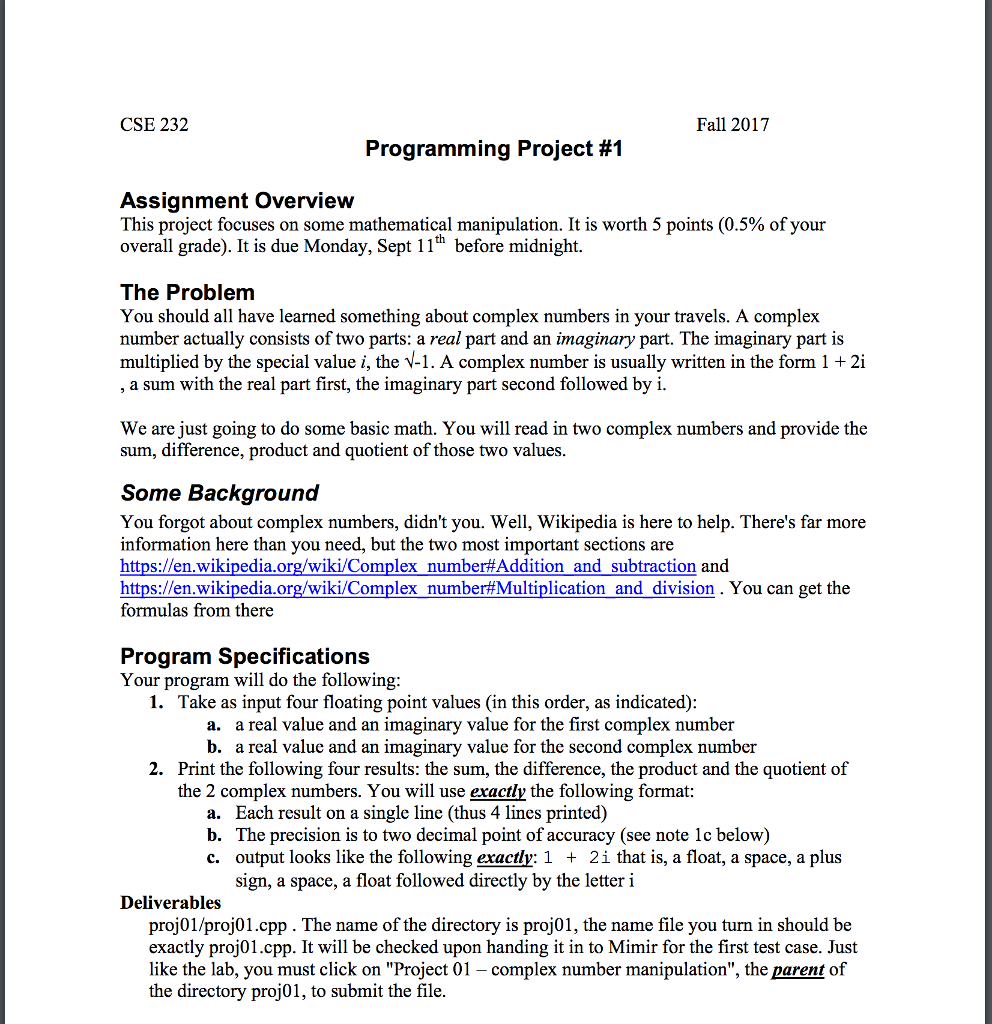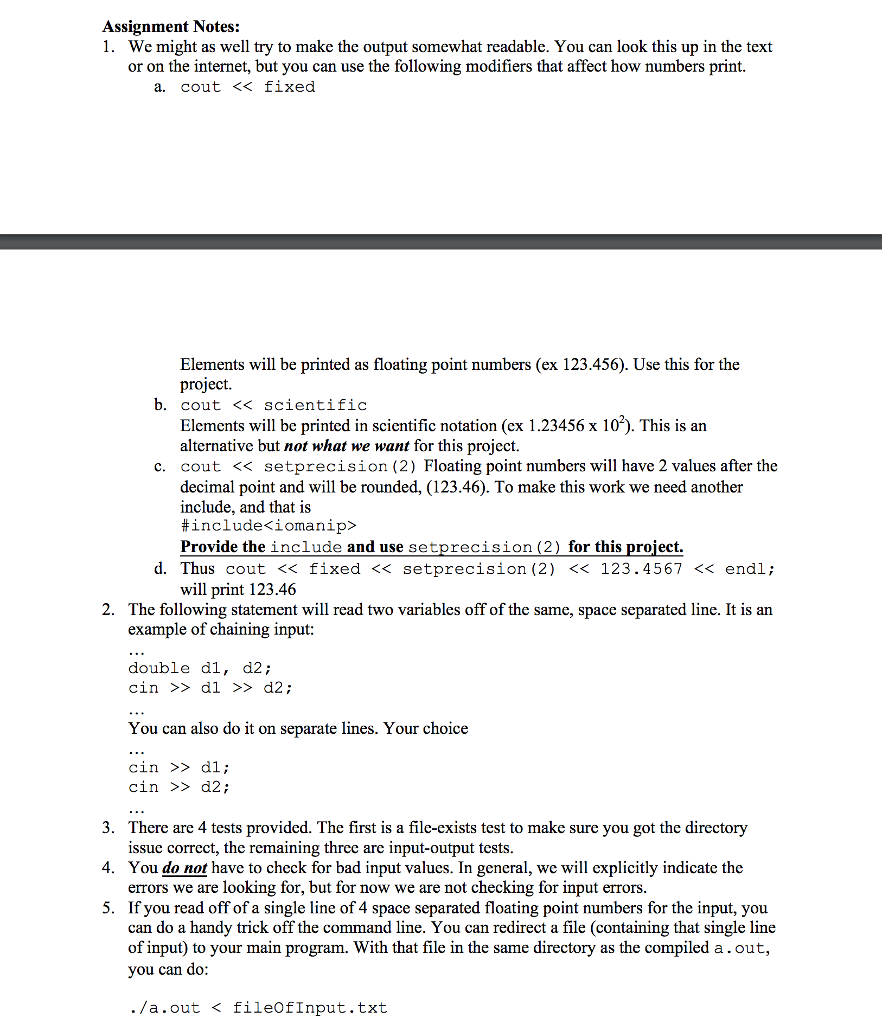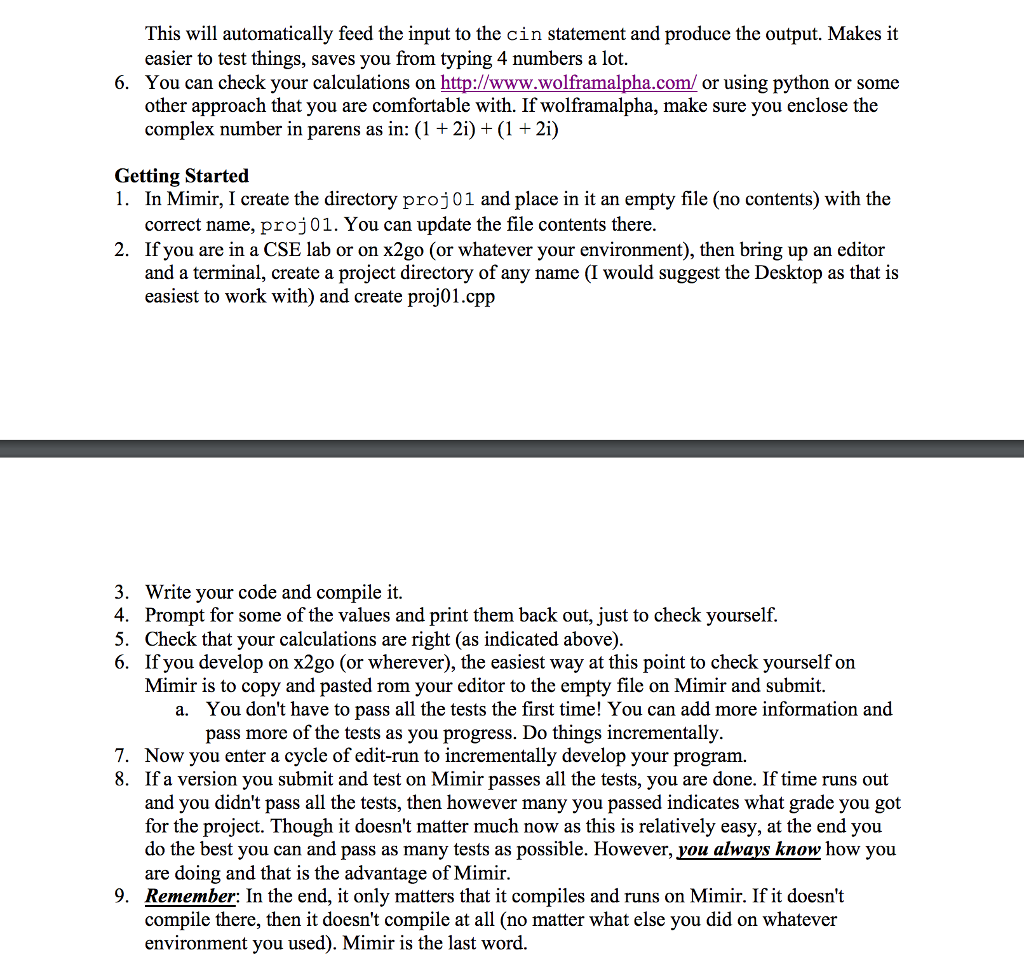I have never worked with C++ before. I someone could help out with this I would greatly appreciate it.

CSE 232 Fall 2017 Programming Project #1 Assignment Overview This project focuses on some mathematical manipulation. It is worth 5 points (0.5% of your overall grade). It is due Monday, Sept 11h before midnight The Problem You should all have learned something about complex numbers in your travels. A complex number actually consists of two parts: a real part and an imaginary part. The imaginary part is multiplied by the special value i, the V-1. A complex number is usually written in the form 1 2i a sum with the real part first, the imaginary part second followed by i We are just going to do some basic math. You will read in two complex numbers and provide the sum, difference, product and quotient of those two values Some Background You forgot about complex numbers, didn’t you. Well, Wikipedia is here to help. There’s far more information here than you need, but the two most important sections are https://en.wikipedia.org/wiki/Complex number#Addition and subtraction and https://en.wikipedia.org/wiki/Complex number#Multiplication and division. You can get the formulas from there Program Specifications Your program will do the following 1. Take as input four floating point values (in this order, as indicated) a. a real value and an imaginary value for the first complex number b. a real value and an imaginary value for the second complex number 2. Print the following four results: the sum, the difference, the product and the quotient of the 2 complex numbers. You will use exactly the following format: a. Each result on a single line (thus 4 lines printed) b. The precision is to two decimal point of accuracy (see note 1c below) c. output looks like the following exactly: 1 + 2i that is, a float, a space, a plus sign, a space, a float followed directly by the letteri Deliverables proj01/proj01.cpp. The name of the directory is proj01, the name file you turn in should be exactly proj01.cpp. It will be checked upon handing it in to Mimir for the first test case. Just like the lab, you must click on “Project 01 – complex number manipulation”, the parent of the directory proj01, to submit the file

//*********proj01.cpp code ******************

//standard input/output streams

#include <iostream>

//library iomanip to print float upto 2 decimal point

#include <iomanip>

//library cmath to calculate power

#include<cmath>

//using namespace below does not require std:: prefix constantly.

using namespace std;

//program start from here from main()

int main()

{

//declare variable r1,i1 to store real and img value of first complex num respectively

float r1,i1;

//declare variable r2,i2 to store real and img value of second complex num respectively

float r2,i2;

//declare variable finalReal, finalImg to store real and img value of final result respectiverly

float finalReal, finalImg;

cout<< “Enter a real value and an imaginary value for the first complex number” << endl;

cin>>r1 >> i1;

cout<<“Enter a real value and an imaginary value for the second complex number” << endl;

cin>>r2 >> i2;

//(r1+i1i)+(r2+i2i)

finalReal=r1+r2;

finalImg=i1+i2;

//fixed << setprecision(2) << finalReal , it print float upto to 2 decimal point

cout<<“Addition: “<< fixed << setprecision(2) << finalReal <<” “<<“+”<<” “<< fixed << setprecision(2) << finalImg<<“i” << endl;

//Subtraction

//(r1+i1i)-(r2+i2i)

finalReal=r1-r2;

finalImg=i1-i2;

cout<<“Substraction: “<< fixed << setprecision(2) << finalReal <<” “<<“+”<<” “<< fixed << setprecision(2) << finalImg<<“i” << endl;

//Multiplication

//(r1+i1i)*(r2+i2i)

finalReal=((r1)*(r2))-((i1)*(i2));

finalImg =((r1)*(i2))+((r2)*(i1));

cout<<“Multiplication: “<< fixed << setprecision(2) << finalReal <<” “<<“+”<<” “<< fixed << setprecision(2) << finalImg<<“i” << endl;

//Division

//(r1+i1i)/(r2+i2i)

finalReal=(((r1)*(r2))+((i1)*(i2)))/(pow(r2,2)+pow(i2,2));

finalImg=(((r2)*(i1))-((r1)*(i2)))/(pow(r2,2)+pow(i2,2));

cout<<“Division: “<< fixed << setprecision(2) << finalReal <<” “<<“+”<<” “<< fixed << setprecision(2) << finalImg<<“i” << endl;

}

//*************input output and screenshot*****************

//*****Test case 1************8

Enter a real value and an imaginary value for the first complex number
2 3
Enter a real value and an imaginary value for the second complex number
3 -4
Substraction: -1.00 + 7.00i
Multiplication: 18.00 + 1.00i
Division: -0.24 + 0.68i

//***Screenshot of above result *****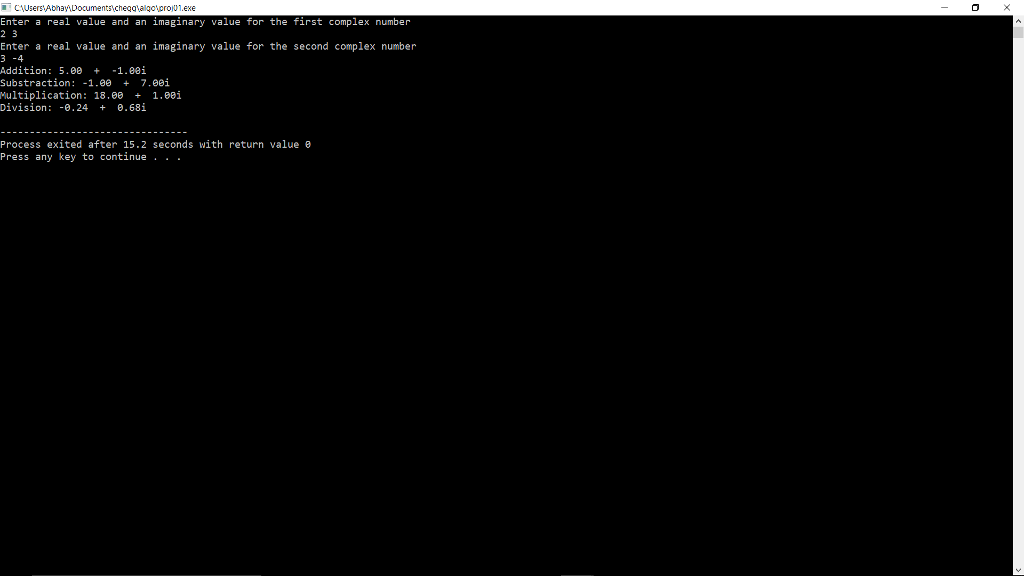//*************Case 2********

Enter a real value and an imaginary value for the first complex number
1 -2
Enter a real value and an imaginary value for the second complex number
-3 4
Substraction: 4.00 + -6.00i
Multiplication: 5.00 + 10.00i
Division: -0.44 + 0.08i

//************Screenshot of above result*******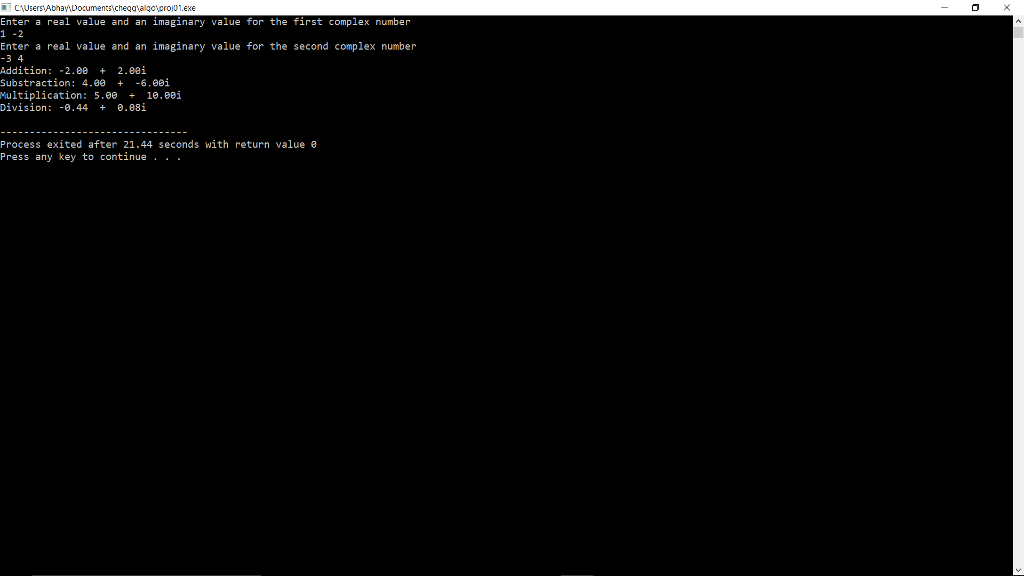Pages (550 words)
Approximate price: -

Help Me Write My Essay - Reasons:Best Online Essay Writing Service

We strive to give our customers the best online essay writing experience. We Make sure essays are submitted on time and all the instructions are followed.Our Writers are Experienced and Professional

Our essay writing service is founded on professional writers who are on stand by to help you any time.Free Revision Fo all Essays

Sometimes you may require our writers to add on a point to make your essay as customised as possible, we will give you unlimited times to do this. And we will do it for free.Timely Essay(s)

We understand the frustrations that comes with late essays and our writers are extra careful to not violate this term. Our support team is always engauging our writers to help you have your essay ahead of time.Customised Essays &100% Confidential

Our Online writing Service has zero torelance for plagiarised papers. We have plagiarism checking tool that generate plagiarism reports just to make sure you are satisfied.Try it now!

## Calculate the price of your order

Total price:
\$0.00

How it works?Fill in the order form and provide all details of your assignment.Proceed with the payment

Choose the payment system that suits you most.HOW OUR ONLINE ESSAY WRITING SERVICE WORKS

Let us write that nagging essay.By clicking on the "PLACE ORDER" button, tell us your requires. Be precise for an accurate customised essay. You may also upload any reading materials where applicable.Pick A & Writer

Our ordering form will provide you with a list of writers and their feedbacks. At step 2, its time select a writer. Our online agents are on stand by to help you just in case.Editing (OUR PART)

At this stage, our editor will go through your essay and make sure your writer did meet all the instructions.